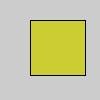Name

| (bitwise OR)

Examplesa = 205  # In binary: 11001101
b = 45  # In binary: 00101101
c = a | b  # In binary: 11101101
print(c)  # Prints "237", the decimal equivalent to 11101101a = 255 << 24  # Binary: 11111111000000000000000000000000
r = 204 << 16  # Binary: 00000000110011000000000000000000
g = 204 << 8   # Binary: 00000000000000001100110000000000
b = 51         # Binary: 00000000000000000000000000110011
# OR the values together: 11111111110011001100110000110011
argb = a | r | g | b
fill(argb)
rect(30, 20, 55, 55)
Description Compares each corresponding bit in the binary representation of the values. For each comparison two 1's yield 1, 1 and 0 yield 1, and two 0's yield 0. This is easy to see when we look at the binary representation of numbers

11010110  # 214
| 01011100  # 92
--------
11011110  # 222

To see the binary representation of a number, use the binary() function with print().
Syntax
value | value2
Parameters
value1 int, char, byte int, char, byte
Related & (bitwise AND)
binary()

Updated on Mon Sep 21 15:53:24 2020.

If you see any errors or have comments, please let us know.